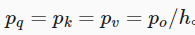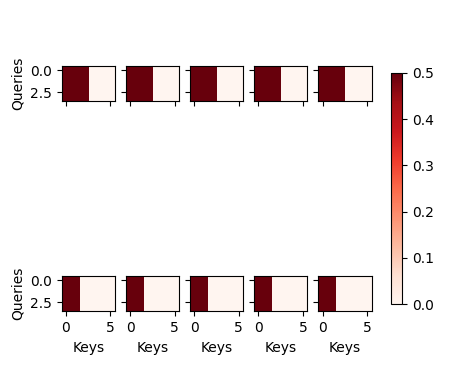## Exercises2

i make a experiments:
i want to add a softmax to the output to check the weights of the output .
the vectof of first vector is [batch_size,0,queries,num_hiddens/num_heads] ,repeate the last dim num_heads, so we can have a vector [batch_size,num_heads,queries,num_hiddens]. then use the softmax weight to measure the weight of the heads.

1 Like

For torch implementation, multi-head attention says it will train “ℎ independently learned linear projections.” However, the implementation for MultiHeadAttention has fixed number of parameters. Anyone can explain why is it? I think it should have ℎ times more parameters than single head attention.

The last dimension of queries is num_hidden, but the input size of w_q is query_size. What if num_hidden is not equal to query_size?

in the implementation, there is a special setting:and Po= num_hiddens.
so for each linear projection, the output dimension should be num_hiddens/h

in the code, it set the linear network to a bigger one, with shape as (query_size, num_hiddens), it means there will be h time of W parameters then a single linear net.

``````self.W_q = nn.Linear(query_size, num_hiddens, bias=bias)
self.W_k = nn.Linear(key_size, num_hiddens, bias=bias)
self.W_v = nn.Linear(value_size, num_hiddens, bias=bias)``````

in the special implementation it sets query_size=k_size=v_size=num_hiddens, which can be found in the attention layer initialization:

transpose_qkv-》transpose_output

Thank you for the reply! I can understand this is a special implementation for running efficiency, but it does make it very hard to understand what is actually happening in a general transformer.

Hi, zppet, thank you for sharing your understanding, however I still have some questions for this section.

We have "pqh=pkh=pvh=po In the following implementation, po is specified via the argument `num_hiddens`", which indicates that qury_size * num_heads = key_size * num_heads = value_size * num_heads = num_hiddens. And we also have num_hiddens = query_size as you mentioned here:

However, this rises an ineuality considered that we define num_heads as 5 (in the following code block).

Could you please explain this point?

Hello,

In the comment of the forward method of MultiHeadAttention, shouldn’t the original queries (/keys/values) shape be (batch_size, no. of queries, query_size), so that when it get multiplied by self.W_q it returns shape (`batch_size`, no. of queries or key-value pairs, `num_hiddens`)?

``````#@save
...

def forward(self, queries, keys, values, valid_lens):
# Shape of `queries`, `keys`, or `values`:
# (`batch_size`, no. of queries or key-value pairs, `num_hiddens`) <------ HERE
# Shape of `valid_lens`:
# (`batch_size`,) or (`batch_size`, no. of queries)
# After transposing, shape of output `queries`, `keys`, or `values`:
# (`batch_size` * `num_heads`, no. of queries or key-value pairs,

...
``````

1 Like

Exercise 1 ：
add one line code this is right? `d2l.show_heatmaps(attention.attention.attention_weights.reshape((batch_size, num_heads, num_queries, num_kvpairs)), xlabel='Keys', ylabel='Queries',figsize=(5, 5))`

and this is the attention weight of each head ?W_q、W_k和W_v不应该是有h个吗，为什么类实现里面就写了一个

The code in this section is so confusing. I made this picure to help myself sort out how the shape of tensor change within multihead attention.